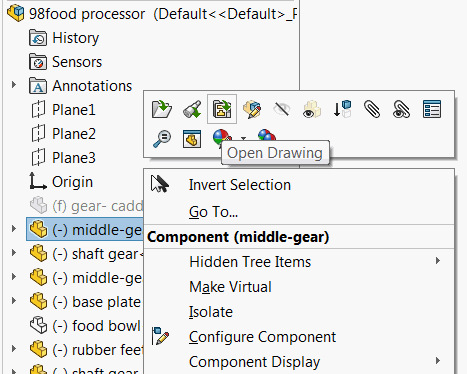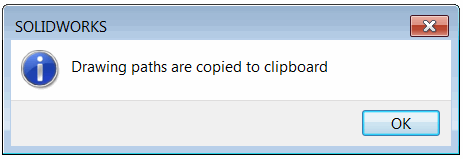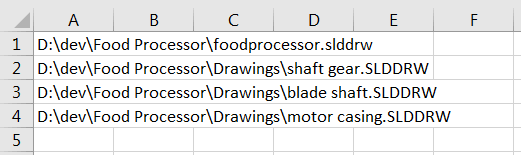# Macro to copy file paths to all drawings of an assembly components using SOLIDWORKS API

More 'Goodies'

This VBA macro finds all the drawings which were created for all components of the active assembly using SOLIDWORKS API and puts the paths to the clipboard.

SOLIDWORKS provides the functionality to open the drawings of the component:Open drawing feature in SOLIDWORKS

This feature allows to find drawings one-by-one, but sometimes it is required to quickly find all drawings used by components of this assembly. This can be a part of automation software. This macro will traverse all the references and find all drawings paths. Once completed the confirmation message below is displayed.Confirmation of completion of drawings extraction operation

The content of the clipboard can be pasted into any text or table editor, like Notepad or Excel (use ctrl+V shortcut or Paste command).Drawing paths copied to Excel

• Suppressed components are excluded from the search
• Drawings are searched in the same folder as the input assembly (including sub folders)
• Drawings are searched by reference, rather than by name, so drawing can have any name
• Drawing paths are separated with a new line symbol

```Dim swApp As SldWorks.SldWorks

Sub main()

Set swApp = Application.SldWorks

Dim swModel As SldWorks.ModelDoc2

try:

On Error GoTo catch

Set swModel = swApp.ActiveDoc

If Not swModel Is Nothing Then

If swModel.GetPathName() = "" Then
Err.Raise vbError, "", "File is not saved"
End If

Dim vDrawingPaths As Variant

Dim dir As String
dir = swModel.GetPathName()
dir = Left(dir, InStrRev(dir, "\"))

If TypeOf swModel Is SldWorks.AssemblyDoc Then
Dim swAssy As SldWorks.AssemblyDoc
Set swAssy = swModel
Dim vRefs As Variant
vRefs = GetAllReferences(swAssy)
vDrawingPaths = GetDrawingsForFiles(vRefs, dir)
ElseIf TypeOf swModel Is SldWorks.PartDoc Then
vDrawingPaths = GetDrawingsForFiles(Array(swModel.GetPathName()), dir)
Else
Err.Raise vbError, "", "Only part or assemblies are supported"
End If

AddPathsToClipboard vDrawingPaths

swApp.SendMsgToUser2 "Drawing paths are copied to clipboard", swMessageBoxIcon_e.swMbInformation, swMessageBoxBtn_e.swMbOk

Else
Err.Raise vbError, "", "Please open part or assembly"
End If

GoTo finally

catch:
Debug.Print Err.Number
swApp.SendMsgToUser2 Err.Description, swMessageBoxIcon_e.swMbStop, swMessageBoxBtn_e.swMbOk

finally:

End Sub

Function GetAllReferences(assy As SldWorks.AssemblyDoc) As Variant

Dim refs() As String
Dim isInit As Boolean
isInit = False

Dim vComps As Variant
vComps = assy.GetComponents(False)

Dim i As Integer

For i = 0 To UBound(vComps)

Dim swComp As SldWorks.Component2

Set swComp = vComps(i)

Dim path As String
path = swComp.GetPathName()

If Not swComp.IsSuppressed() Then
If Not isInit Then
isInit = True
ReDim refs(0)
refs(0) = path
Else
If Not ContainsFilePath(refs, path) Then
ReDim Preserve refs(UBound(refs) + 1)
refs(UBound(refs)) = path
End If
End If
End If

Next

GetAllReferences = refs

End Function

Function GetDrawingsForFiles(files As Variant, path As String) As Variant

Dim drawingPaths() As String
Dim isInit As Variant
isInit = False

Dim vAllDrawings As Variant
vAllDrawings = FindAllDrawings(path)

If Not IsEmpty(vAllDrawings) Then

Dim i As Integer

For i = 0 To UBound(vAllDrawings)

Dim drawPath As String
drawPath = vAllDrawings(i)

Dim vDeps As Variant

vDeps = swApp.GetDocumentDependencies2(drawPath, True, True, False)
Dim j As Integer

If Not IsEmpty(vDeps) Then

For j = 1 To UBound(vDeps) Step 2
If ContainsFilePath(files, CStr(vDeps(j))) Then
If Not isInit Then
isInit = True
ReDim drawingPaths(0)
Else
ReDim Preserve drawingPaths(UBound(drawingPaths) + 1)
End If
drawingPaths(UBound(drawingPaths)) = drawPath
Exit For
End If
Next

End If

Next

End If

GetDrawingsForFiles = drawingPaths

End Function

Function FindAllDrawings(path As String) As Variant

Const DRAW_EXTENSION As String = "slddrw"
FindAllDrawings = GetFiles(path, True, DRAW_EXTENSION)

End Function

Function GetFiles(path As String, Optional includeSubFolders As Boolean = True, Optional ext As String = "") As Variant

Dim paths() As String
Dim isInit As Boolean

isInit = False

Dim fso As Object
Set fso = CreateObject("Scripting.FileSystemObject")

Dim folder As Object
Set folder = fso.GetFolder(path)

CollectFilesFromFolder folder, includeSubFolders, ext, paths, isInit

If isInit Then
GetFiles = paths
Else
GetFiles = Empty
End If

End Function

Sub CollectFilesFromFolder(folder As Object, includeSubFolders As Boolean, ext As String, ByRef paths() As String, ByRef isInit As Boolean)

For Each file In folder.files
Dim fileExt As String
fileExt = Right(file.path, Len(file.path) - InStrRev(file.path, "."))
If LCase(fileExt) = LCase(ext) Then
If Not isInit Then
ReDim paths(0)
isInit = True
Else
ReDim Preserve paths(UBound(paths) + 1)
End If
paths(UBound(paths)) = file.path
End If
Next

If includeSubFolders Then
Dim subFolder As Object
For Each subFolder In folder.SubFolders
CollectFilesFromFolder subFolder, includeSubFolders, ext, paths, isInit
Next
End If

End Sub

Sub AddPathsToClipboard(vPaths As Variant)

Dim text As String
Dim i As Integer

For i = 0 To UBound(vPaths)
If i <> 0 Then
text = text & vbCrLf
End If
text = text & CStr(vPaths(i))
Next

Dim dataObject As Object
Set dataObject = CreateObject("new:{1C3B4210-F441-11CE-B9EA-00AA006B1A69}")
dataObject.SetText text
dataObject.PutInClipboard
Set dataObject = Nothing

End Sub

Function ContainsFilePath(arr As Variant, item As String) As Boolean

Dim i As Integer

For i = 0 To UBound(arr)
If LCase(arr(i)) = LCase(item) Then
ContainsFilePath = True
Exit Function
End If
Next

Contains = False

End Function
```